# Please show all work A student set up a magnetic optical bench with a 5.0-cm focal...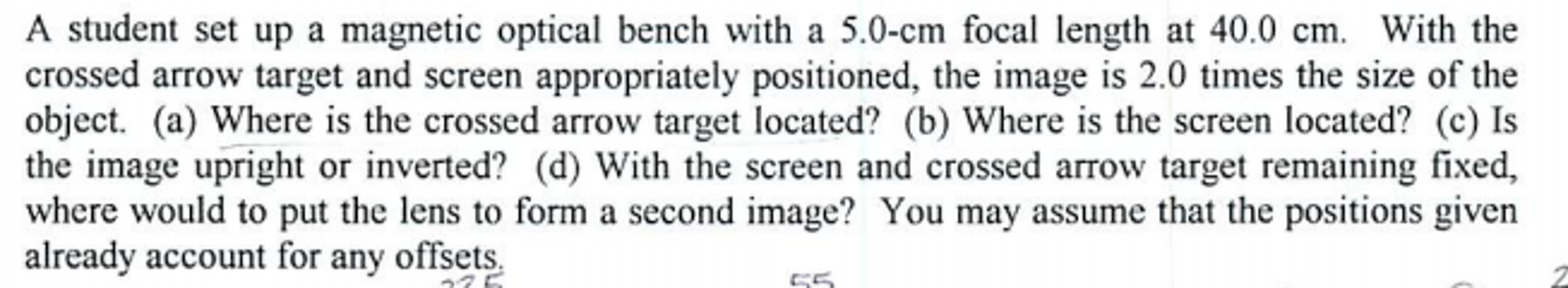A student set up a magnetic optical bench with a 5.0-cm focal length at 40.0 cm. With the crossed arrow target and screen appropriately positioned, the image is 2.0 times the size of the object, (a) Where is the crossed arrow target located? (b) Where is the screen located? (c) Is the image upright or inverted? (d) With the screen and crossed arrow target remaining fixed, where would to put the lens to form a second image? You may assume that the positions given already account for any offsets.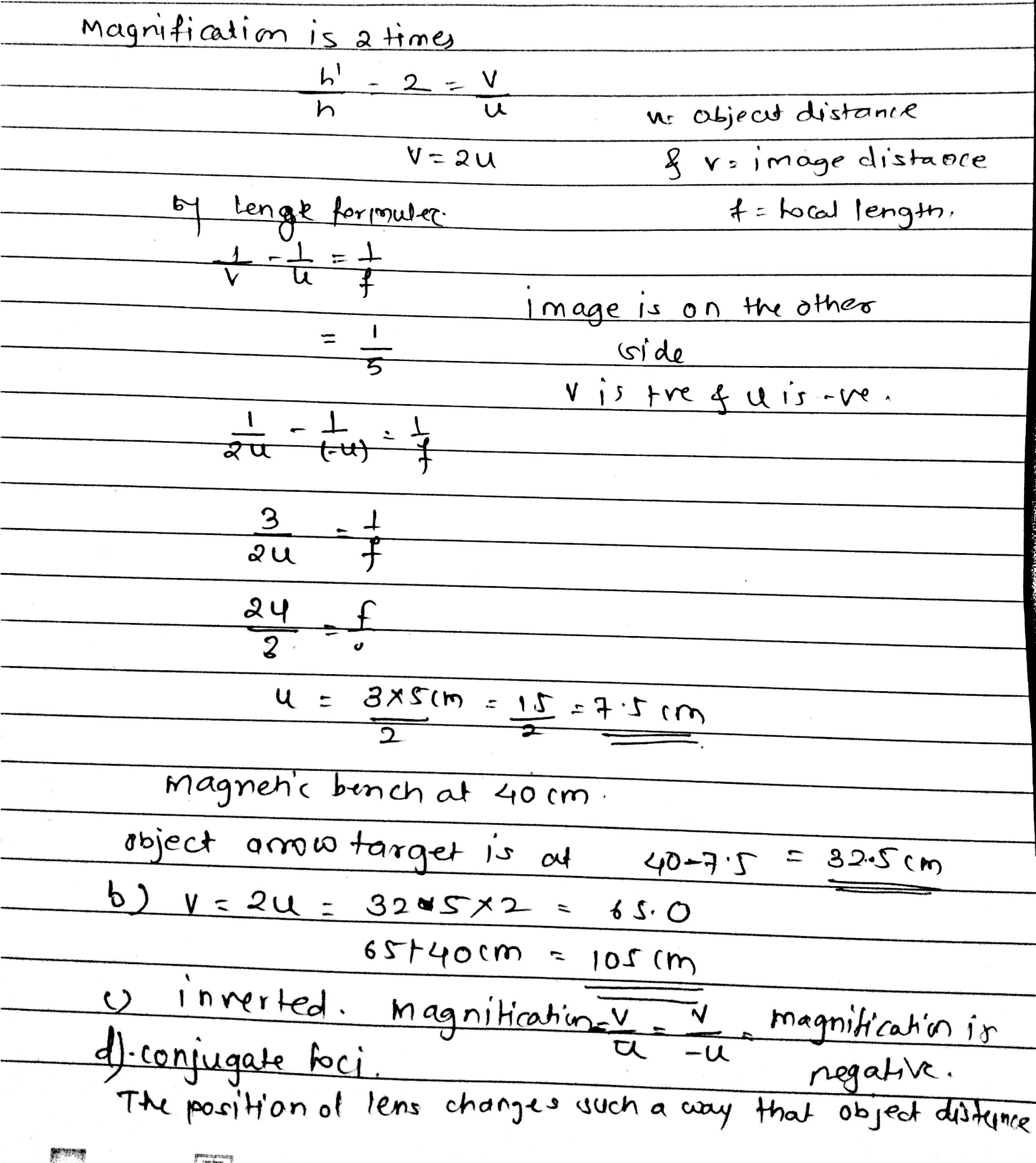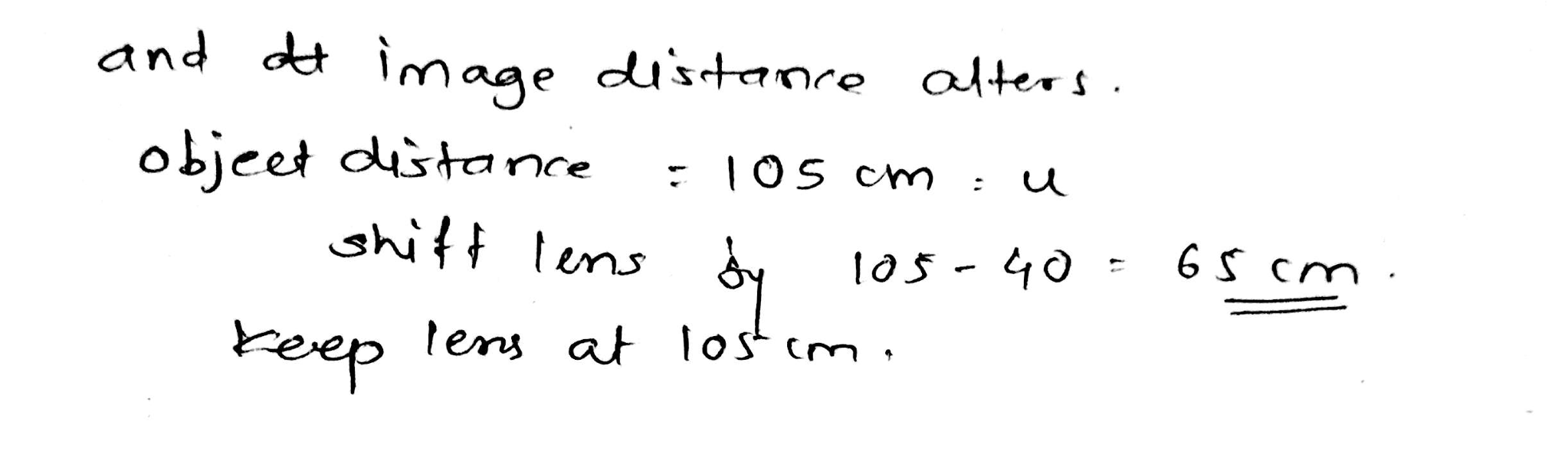#### Earn Coin

Coins can be redeemed for fabulous gifts.

Similar Homework Help Questions
• ### A student set up a magnetic optical bench as you saw in the video. You may...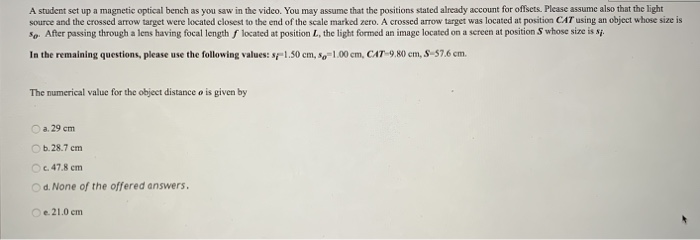A student set up a magnetic optical bench as you saw in the video. You may assume that the positions stated already account for offsets. Please assume also that the light source and the crossed arrow target were located closest to the end of the scale marked zero. A crossed arrow target was located at position CAT using an object whose size is So. After passing through a lens having focal length located at position L, the light formed an...

• ### Magnetic optical bench problem

A student set up the magnetic optical bench as shown. The locations of the component holders for the crossed arrow target (CAT), and lens (L), are as follows,respectively: 25.8 cm, 42.6 cm. The offsets for the CAT and the S are 3.0 mm, and the offset for L is 6.0 mm. The image formed on the screen is twice the size of theobject. (a) Calculate the position of the component holder of the screen. (b) Calculate the focal length of...

• ### Question Completion Status: Question 5 1 points Save Answer A student set up a magnetic optical...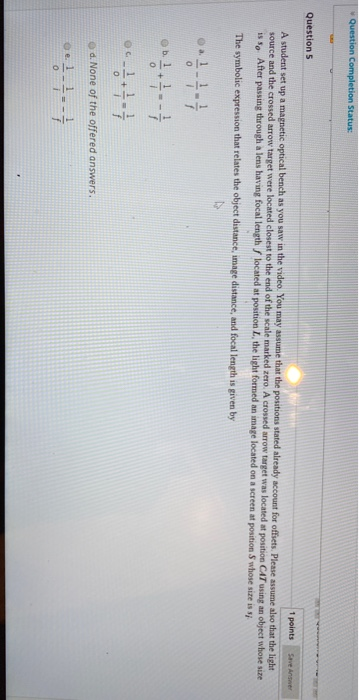Question Completion Status: Question 5 1 points Save Answer A student set up a magnetic optical bench as you saw in the video. You may assume that the positions stated already account for offsets. Please assume also that the light source and the crossed arrow target were located closest to the end of the scale marked zero. A crossed arrow target was located at position CAT using an object whose sire is so After passing through a lens having focal...

• ### A converging lens of 15 cm focal length is placed on an optical bench that is...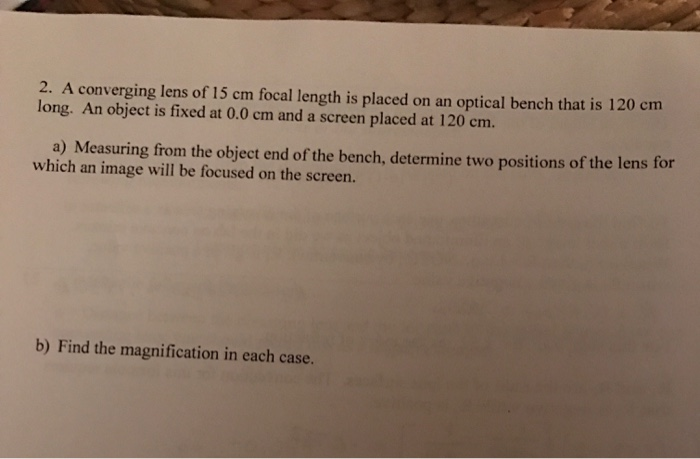A converging lens of 15 cm focal length is placed on an optical bench that is 120 cm long. An object is fixed at 0.0 cm and a screen placed at 120 cm. a) Measuring from the object end of the bench, determine two positions of the lens for which an image will be focused on the screen. b) Find the magnification in each case.

• ### A lens focal length f=+35cm is located on an optical bench. An object is located 50.0cm...

A lens focal length f=+35cm is located on an optical bench. An object is located 50.0cm in front of the lens. Determine the image position for this system. State whether the image is real or virtual. Upright or inverted. Magnified or demagnified.

• ### A converging lens of 15cm focal length is placed on an optical bench that is 120cm long. An object fixed at 0.0cm and s...

A converging lens of 15cm focal length is placed on an optical bench that is 120cm long. An object fixed at 0.0cm and screen is placed at 140.0 cm. Measuring from the object end of the bench, determine the two positions of the lens for which an image will be focused on the screen. Find all magnification in each case.

• ### The type of lens is not given. (10%) Problem 6: Two lenses are mounted d= 29 cm apart on an optical bench. The focal len...The type of lens is not given. (10%) Problem 6: Two lenses are mounted d= 29 cm apart on an optical bench. The focal length of the first lens is fı = 7.9 cm and that of the second lens is f2 = 5.6 cm. An object of height ho = 3.4 cm is placed at a distance of do = 29 cm in front of the first lens. 25% Part (a) Ignoring the second lens for now, at what...

• ### Two converging lenses having focal lengths off, = 11.3 cm and f, - 20.0 cm are...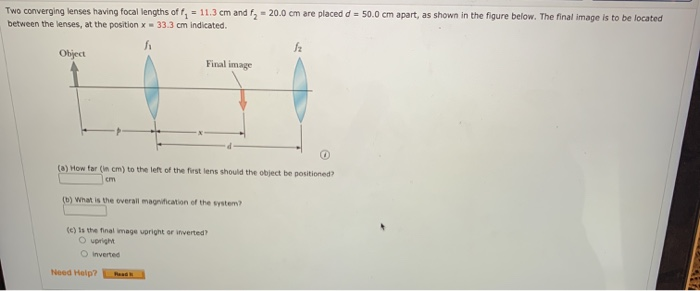Two converging lenses having focal lengths off, = 11.3 cm and f, - 20.0 cm are placed d = 50.0 cm apart, as shown in the figure below. The final image is to be located between the lenses, at the position x = 33.3 cm Indicated. 12 Object Final image (5) How far (in cm) to the left of the first lens should the object be positioned? cm (b) What is the overall magnification of the system? (c) is the...

• ### A converging lens with a focal length of 4.2 cm is located 20.7 cm to the...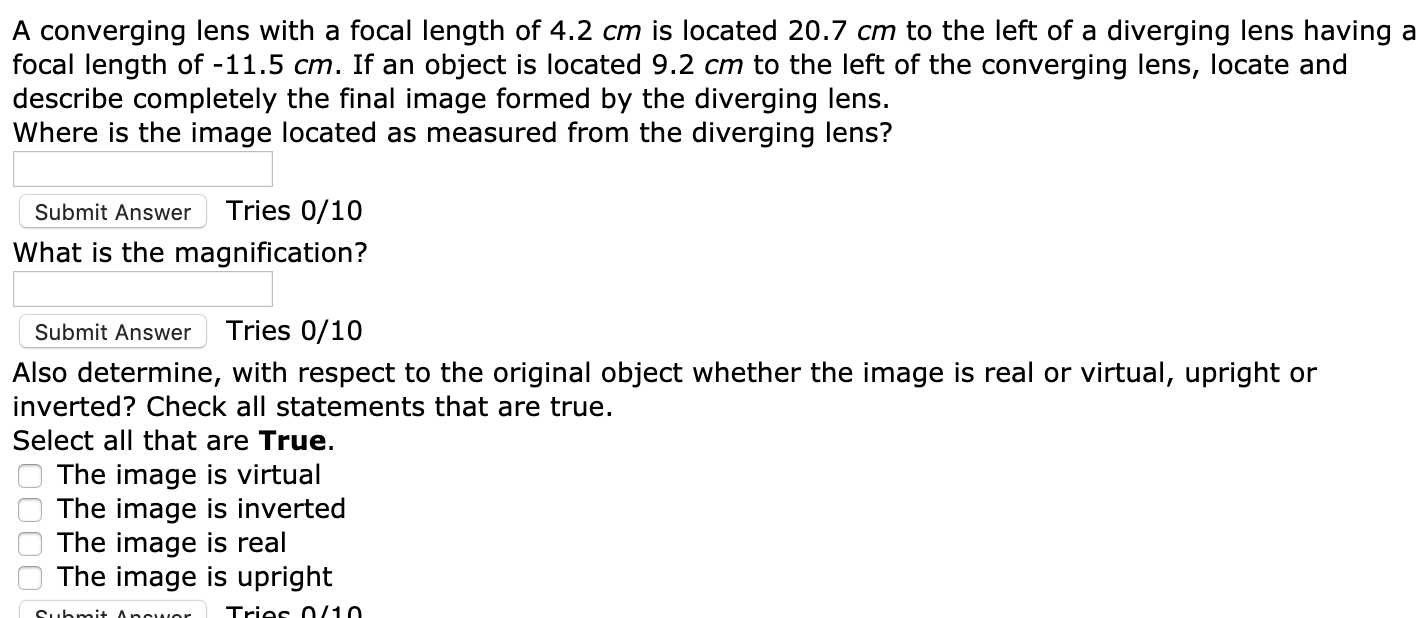A converging lens with a focal length of 4.2 cm is located 20.7 cm to the left of a diverging lens having a focal length of -11.5 cm. If an object is located 9.2 cm to the left of the converging lens, locate and describe completely the final image formed by the diverging lens. Where is the image located as measured from the diverging lens? Submit Answer Tries 0/10 What is the magnification? Submit Answer Tries 0/10 Also determine, with...

• ### A converging lens with a focal length of 6.0 cm is located 24.0 cm to the...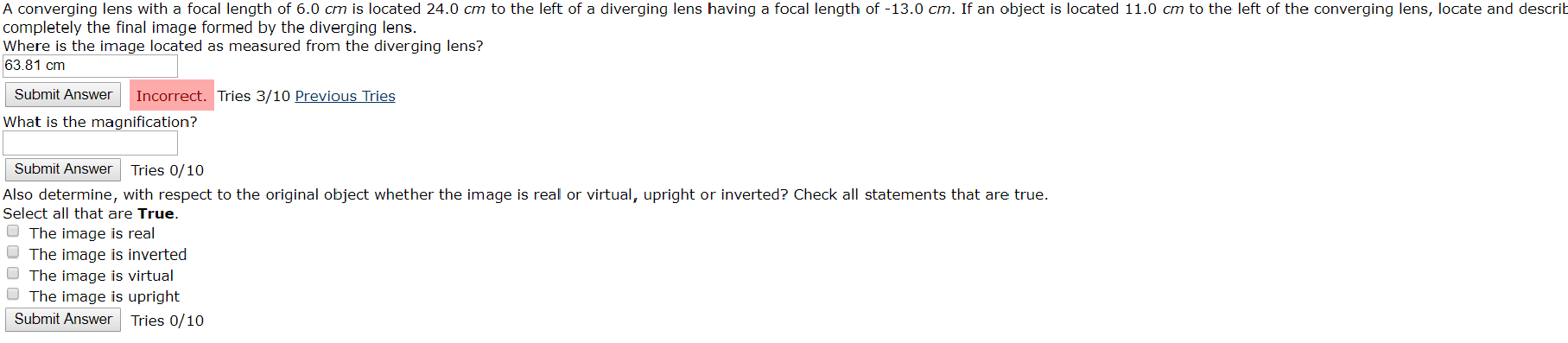A converging lens with a focal length of 6.0 cm is located 24.0 cm to the left of a diverging lens having a focal length of -13.0 cm. If an object is located 11.0 cm to the left of the converging lens, locate and describ completely the final image formed by the diverging lens. Where is the image located as measured from the diverging lens? 63.81 cm Submit Answer Incorrect. Tries 3/10 Previous Tries What is the magnification? Submit Answer...

Free Homework App